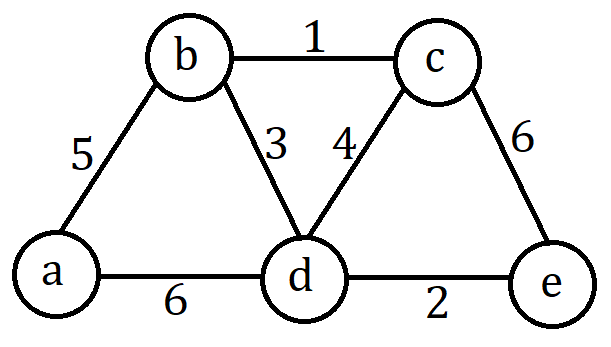# Design And Analysis Of Algorithm 2018 – BSc Computer Science Part 3

#### Paper code: 13524 1524 B.Sc. (Computer Science) (Part 3) Examination, 2018 Paper No. 2.3 DESIGN AND ANALYSIS OF ALGORITHM

##### Time: Three Hours] [Maximum Marks: 50

Note: Attempt all the five questions. All questions carry equal marks.

1. Attempt any two parts of the following:

(a) Give the general strategy of Divide and Conquer method.

(b) Derive the worst case analysis of Merge Sort using suitable illustrations.

(c) Define the single source shortest path problem.

2. Attempt any two parts of the following:

(a) Give the algorithm for finding the number of binary digits in n’s Binary representation, where n is a positive decimal integer. Find the recurrence relation and complexity.

(b) Write down the properties of asymptotic notations.

(c) Compare the orders of growth of  and .

3. Attempt any two parts of the following:

(a) State and explain the Merge Sort algorithm and give the recurrence relation and efficiency.

(b) Write the recursive Fibonacci algorithm and its recurrence relation.

(c) Explain the method used for performing multiplication two large integers. How Divide and Conquer method can be used to solve the same?

4. Attempt any two parts of the following:

(a) List out the memory functions used under dynamic programming.

(b) Write the Heap Sort algorithm and estimate its running time.

(c) Apply Kruskal’s algorithm to find a minimum spanning tree of the following graph:5. Write short notes on any two of the following:

1. 8-Queens Problems
2. Breadth First Search
3. Graph Coloring
4. Travelling Salesperson Problem

………………END………………#### Lokesh Kumar

Being EASTER SCIENCE's founder, Lokesh Kumar wants to share his knowledge and ideas. His motive is "We assist you to choose the best", He believes in different thinking.

This site uses Akismet to reduce spam. Learn how your comment data is processed.